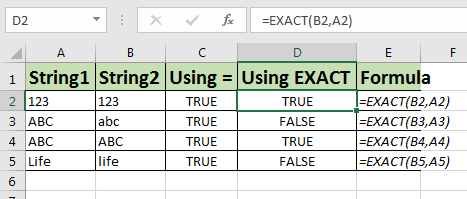# How to Compare Text in Microsoft Excel

Let’s learn how to compare two strings in Excel. To compare two strings we can use comparison operator “=”. It will work fine until you want to do a case sensitive comparison.To compare two string with case sensitivity we will use Excel EXACT function.

Exact function compares two strings and returns TRUE only if they are exactly same. It keeps in account Upper Case and Lower Case alphabets.
Generic Formula

=EXACT(string1, string2)

string1, string2: The string you want to compare.

Case Sensitive Comparison String Example
If in cell A2 you have string “ABC” and in B2 “ABc” then below formula will return FALSE.

=EXACT(A2, B2)

Where this “=A2=B2” will return TRUE.

So yeah guys, you can compare stings with case sensitivity in excel using EXACT function easily.

Popular Articles:

50 Excel Shortcuts to Increase Your Productivity

How to use the VLOOKUP Function in Excel

How to use the COUNTIF function in Excel

How to use the SUMIF Function in Excel

Terms and Conditions of use

The applications/code on this site are distributed as is and without warranties or liability. In no event shall the owner of the copyrights, or the authors of the applications/code be liable for any loss of profit, any problems or any damage resulting from the use or evaluation of the applications/code.